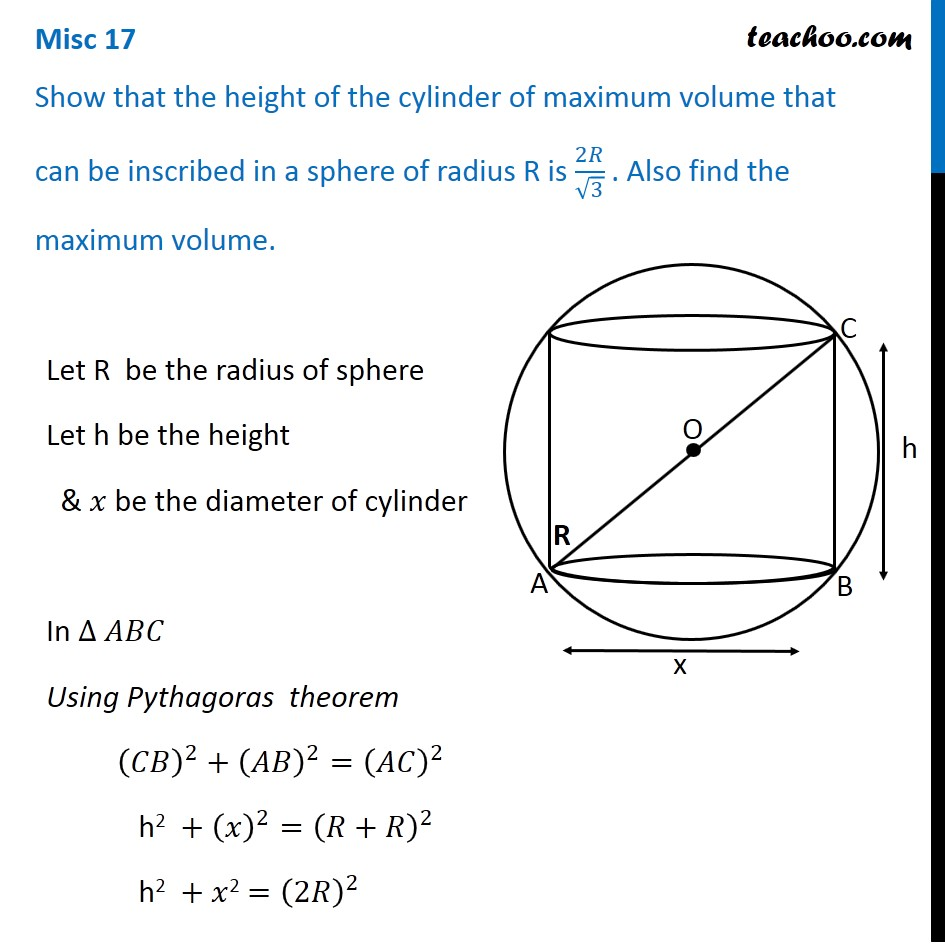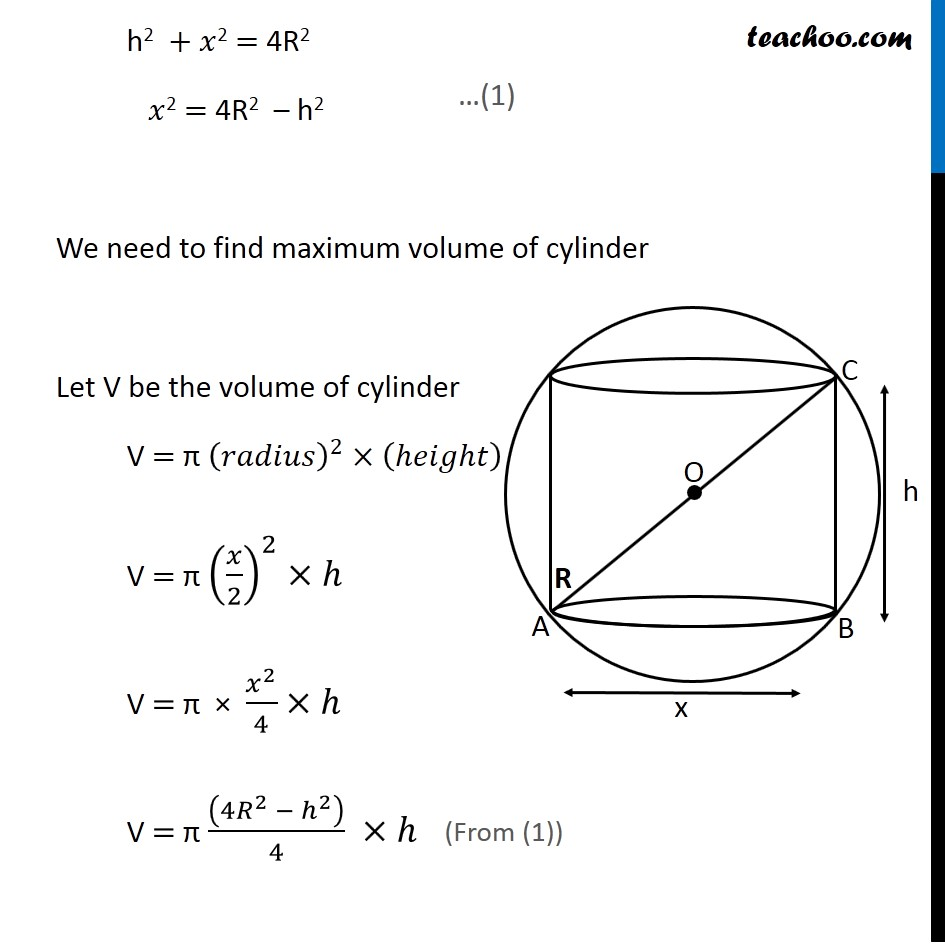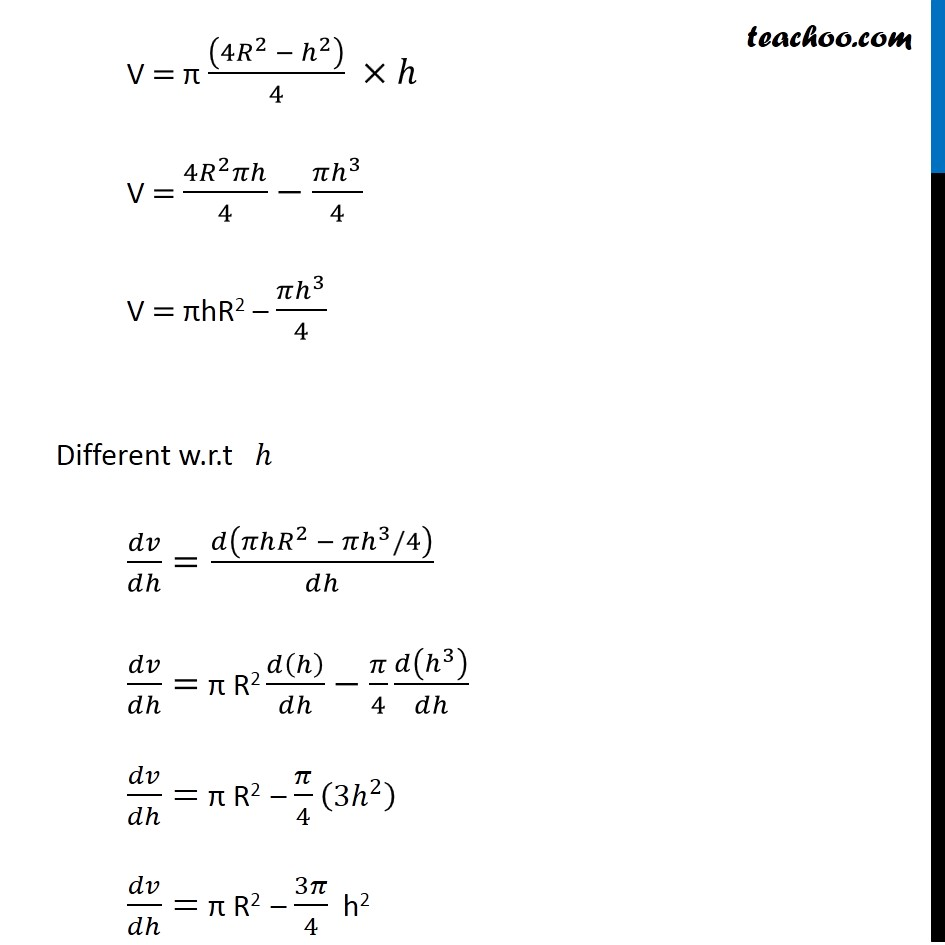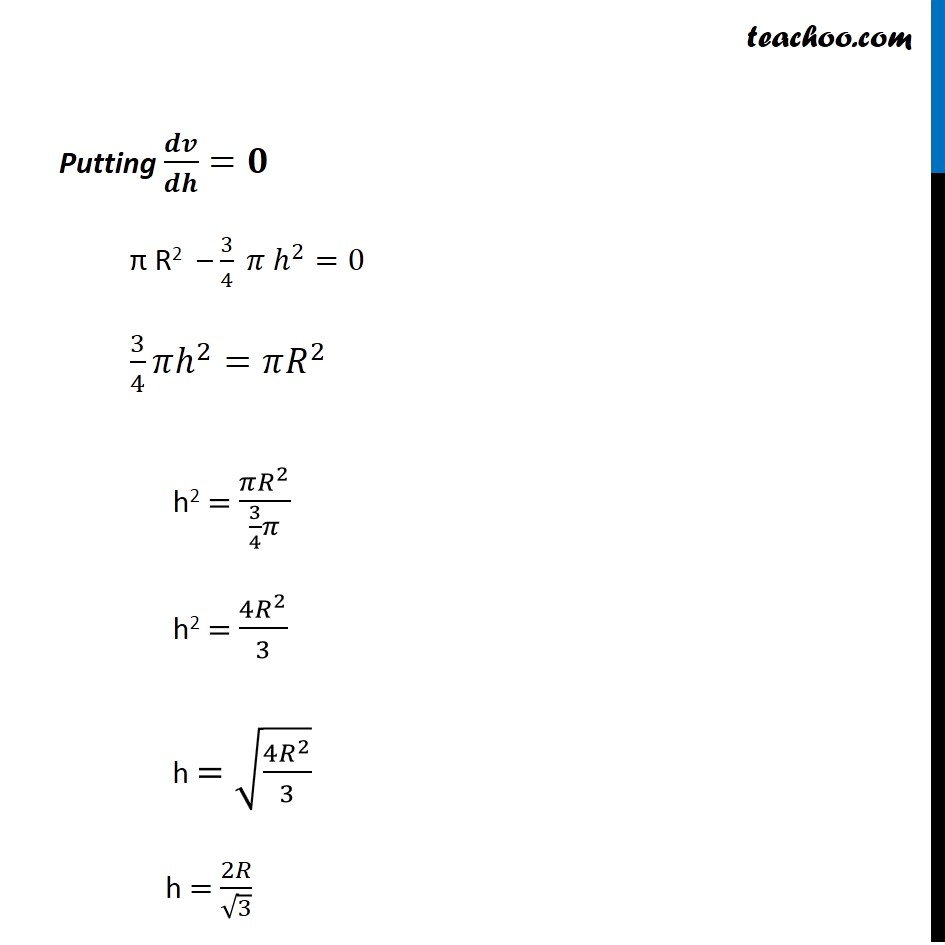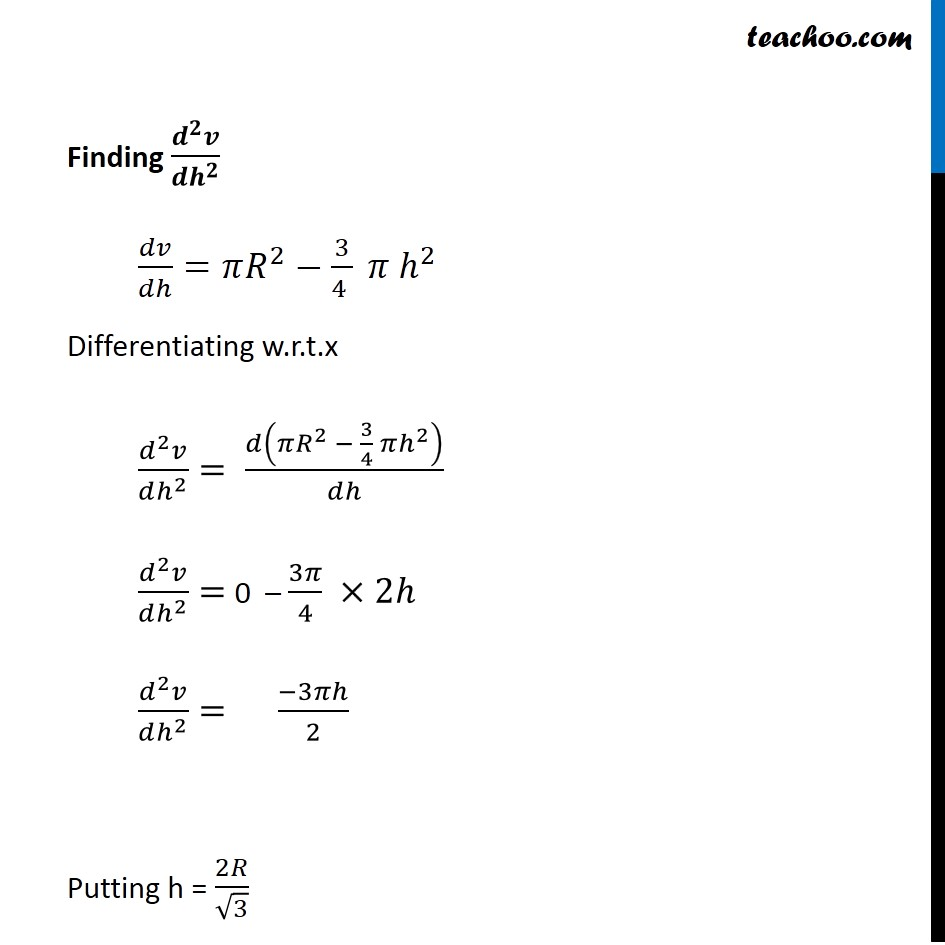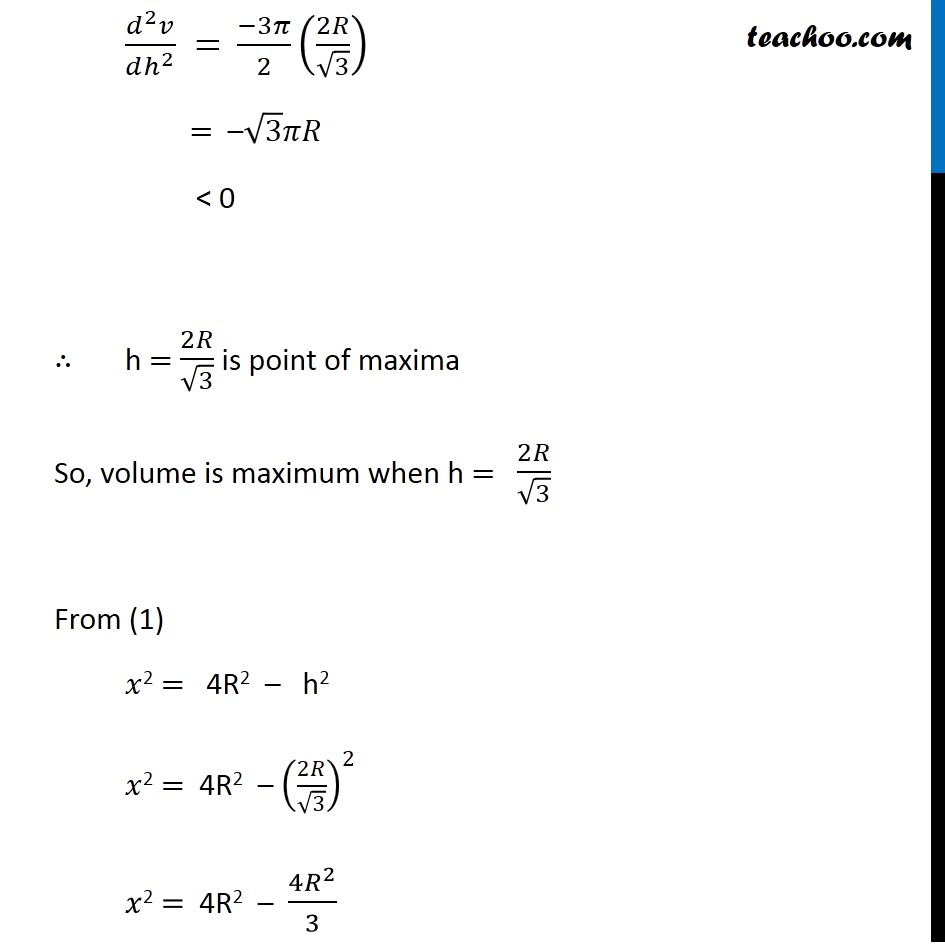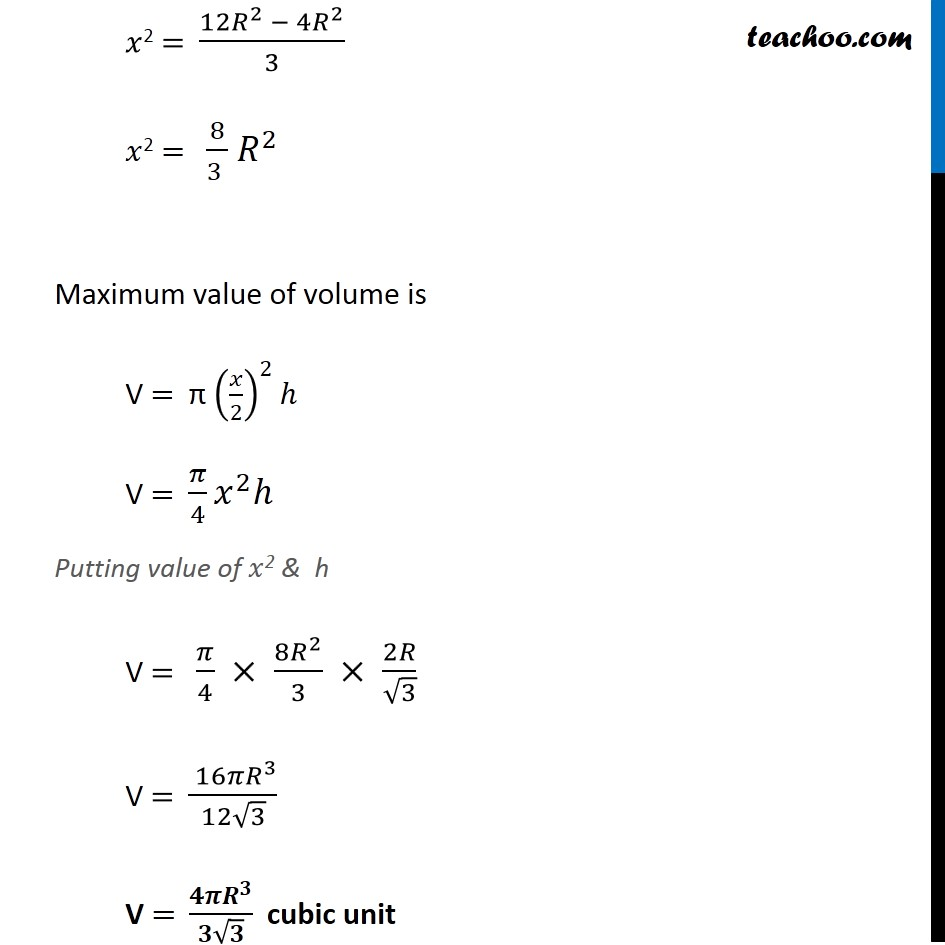Subscribe to our Youtube Channel - https://you.tube/teachoo

1. Chapter 6 Class 12 Application of Derivatives
2. Serial order wise
3. Miscellaneous

Transcript

Misc 17 Show that the height of the cylinder of maximum volume that can be inscribed in a sphere of radius R is 2𝑅/√3 . Also find the maximum volume. Let R be the radius of sphere Let h be the height & 𝑥 be the diameter of cylinder In ∆ 𝐴𝐵𝐶 Using Pythagoras theorem (𝐶𝐵)^2+(𝐴𝐵)^2=(𝐴𝐶)^2 h2 + (𝑥)^2=(𝑅+𝑅)^2 h2 + 𝑥2 =(2𝑅)^2 h2 + 𝑥2 = 4R2 𝑥2 = 4R2 – h2 We need to find maximum volume of cylinder Let V be the volume of cylinder V = π (𝑟𝑎𝑑𝑖𝑢𝑠)^2×(ℎ𝑒𝑖𝑔ℎ𝑡) V = π (𝑥/2)^2×ℎ V = π × 𝑥^2/4×ℎ V = π ((4𝑅^2 − ℎ^2 ))/4 ×ℎ …(1) V = π ((4𝑅^2 − ℎ^2 ))/4 ×ℎ V = (4𝑅^2 𝜋ℎ)/4−(𝜋ℎ^3)/4 V = πhR2 – (𝜋ℎ^3)/4 Different w.r.t ℎ 𝑑𝑣/𝑑ℎ=𝑑(𝜋ℎ𝑅^2 − 𝜋ℎ^3/4)/𝑑ℎ 𝑑𝑣/𝑑ℎ= π R2 𝑑(ℎ)/𝑑ℎ−𝜋/4 𝑑(ℎ^3 )/𝑑ℎ 𝑑𝑣/𝑑ℎ= π R2 – 𝜋/4 (3ℎ^2 ) 𝑑𝑣/𝑑ℎ= π R2 – 3𝜋/4 h2 Putting 𝒅𝒗/𝒅𝒉=𝟎 π R2 – 3/4 𝜋 ℎ^2=0 3/4 𝜋ℎ^2=𝜋𝑅^2 h2 = (𝜋𝑅^2)/(3/4 𝜋) h2 = (4𝑅^2)/3 h =√((4𝑅^2)/3) h = 2𝑅/√3 Finding (𝒅^𝟐 𝒗)/(𝒅𝒉^𝟐 ) 𝑑𝑣/𝑑ℎ=𝜋𝑅^2−3/(4 ) 𝜋 ℎ^2 Differentiating w.r.t.x (𝑑^2 𝑣)/(𝑑ℎ^2 )= 𝑑(𝜋𝑅^2 − 3/4 𝜋ℎ^2 )/𝑑ℎ (𝑑^2 𝑣)/(𝑑ℎ^2 )= 0 – 3𝜋/4 ×2ℎ (𝑑^2 𝑣)/(𝑑ℎ^2 )= (−3𝜋ℎ)/2 Putting h = 2𝑅/√3 (𝑑^2 𝑣)/(𝑑ℎ^2 ) = (−3𝜋)/2 (2𝑅/√3) = –√3𝜋𝑅 < 0 ∴ h = 2𝑅/√3 is point of maxima So, volume is maximum when h = 2𝑅/√3 From (1) 𝑥2 = 4R2 – h2 𝑥2 = 4R2 – (2𝑅/√3)^2 𝑥2 = 4R2 – (4𝑅^2)/3 𝑥2 = (12𝑅^2 − 4𝑅^2)/3 𝑥2 = 8/(3 ) 〖 𝑅〗^2 Maximum value of volume is V = π (𝑥/2)^2 ℎ V = 𝜋/4 𝑥^2 ℎ Putting value of 𝑥2 & h V = 𝜋/4 × (8𝑅^2)/3 × 2𝑅/√3 V = ( 16𝜋𝑅^3)/(12√3) V = (𝟒𝝅𝑹^𝟑)/(𝟑√𝟑) cubic unit

Miscellaneous